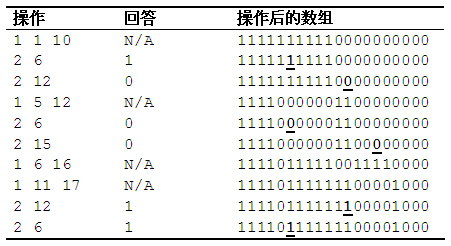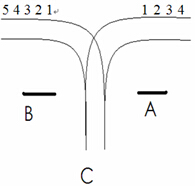# 「POJ-1741」tree-点分治

Give a tree with n vertices,each edge has a length(positive integer less than 1001).
Define dist(u,v)=The min distance between node u and v.
Give an integer k,for every pair (u,v) of vertices is called valid if and only if dist(u,v) not exceed k.
Write a program that will count how many pairs which are valid for a given tree.

# 「BZOJ 1251」序列终结者 - Splay

1. [L,R] 这个区间内的所有数加上 V
2. [L,R] 这个区间翻转，比如 1 2 3 4 变成 4 3 2 1
3. [L,R] 这个区间中的最大值。 最开始所有元素都是 0

# 「NOIP2016」蚯蚓-单调队列

$m$ 秒内，每一秒被切断的蚯蚓被切断前的长度（有 $m$ 个数）；
$m$ 秒后，所有蚯蚓的长度（有 $n + m$ 个数）。

# 「CQOI 2006」简单题

## 简单题

CQOI2006 T1

### 题目描述# 「SuperOJ 405」系列操作II

## 系列操作Ⅱ

### 题目描述

1. $a_l, a_{ l + 1 }, \cdots, a_r(1 \leq l \leq r \leq n)$ 加上 $x(-10 ^ 3 \leq x \leq 10 ^ 3)$
2. 求 $a_i(1 \leq i \leq n)$

### 输入格式

0 1 r x ，表示 $a_l, a_{l+1},\cdots,a_r$ 加上 $x$。

1 i ，求 $a_i$。

# 「SuperOJ 404」系列操作I

## 系列操作Ⅰ

### 题目描述

1. $a_i(1 \leq i \leq n)$ 加上 $x(-10^3 \leq x \leq 10^3)$
2. 求 $max { a_l, a_{l+1}, \cdots ,a_r } (1 \leq l \leq r \leq n)$

### 输入格式

0 i x，表示 $a_i$ 加上 $x$。

1 l r，求 $max{ a_l,a_{l+1}, \cdots, a_r }$。

# 「SuperOJ 142」车厢调度

## 车厢调度

### 题目描述# 「SuperOJ148」表达式括号匹配

## 表达式括号匹配

### 输出格式

###### Your browser is out-of-date!

Update your browser to view this website correctly. Update my browser now

×